5129

Mathematics Integration Level: Misc Level

Integerate the following by parts by using ILATE.
I = inverse trigonometric
L = logarithmic
A = algebraic
T = trigonometric
E = exponential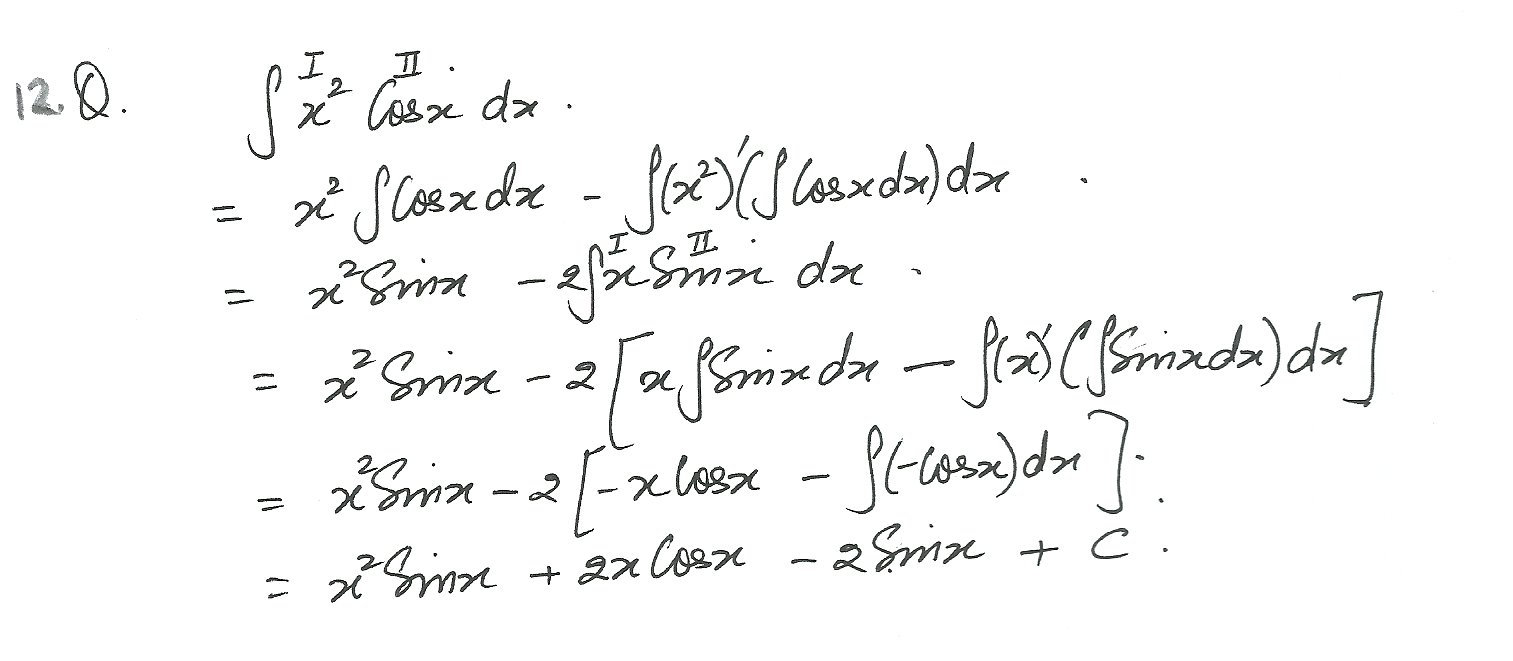5128

Mathematics Integration Level: Misc Level

Integerate the following by parts by using ILATE.
I = inverse trigonometric
L = logarithmic
A = algebraic
T = trigonometric
E = exponential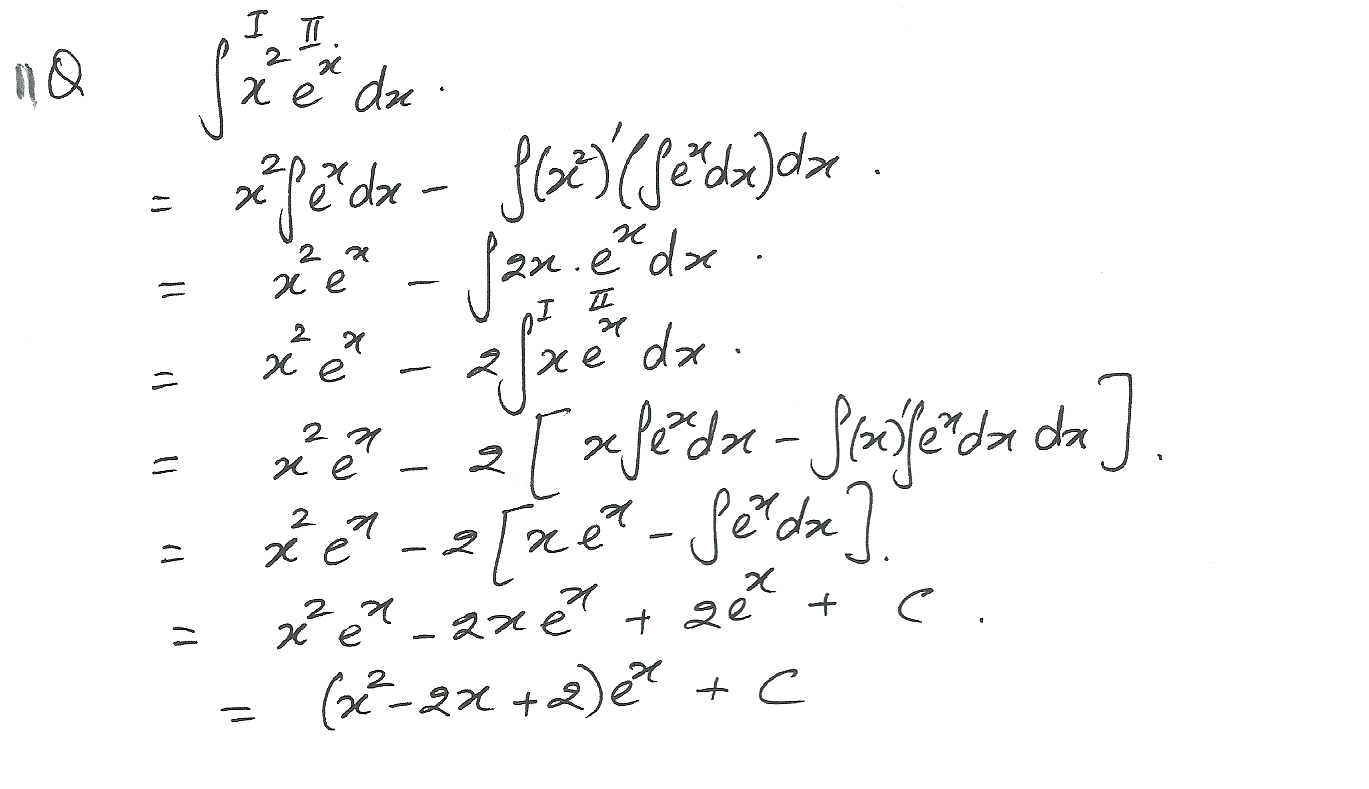5127

Mathematics Integration Level: Misc Level

Integerate the following by parts by using ILATE.
I = inverse trigonometric
L = logarithmic
A = algebraic
T = trigonometric
E = exponential5126

Mathematics Integration Level: Misc Level

Integerate the following by parts by using ILATE.
I = inverse trigonometric
L = logarithmic
A = algebraic
T = trigonometric
E = exponential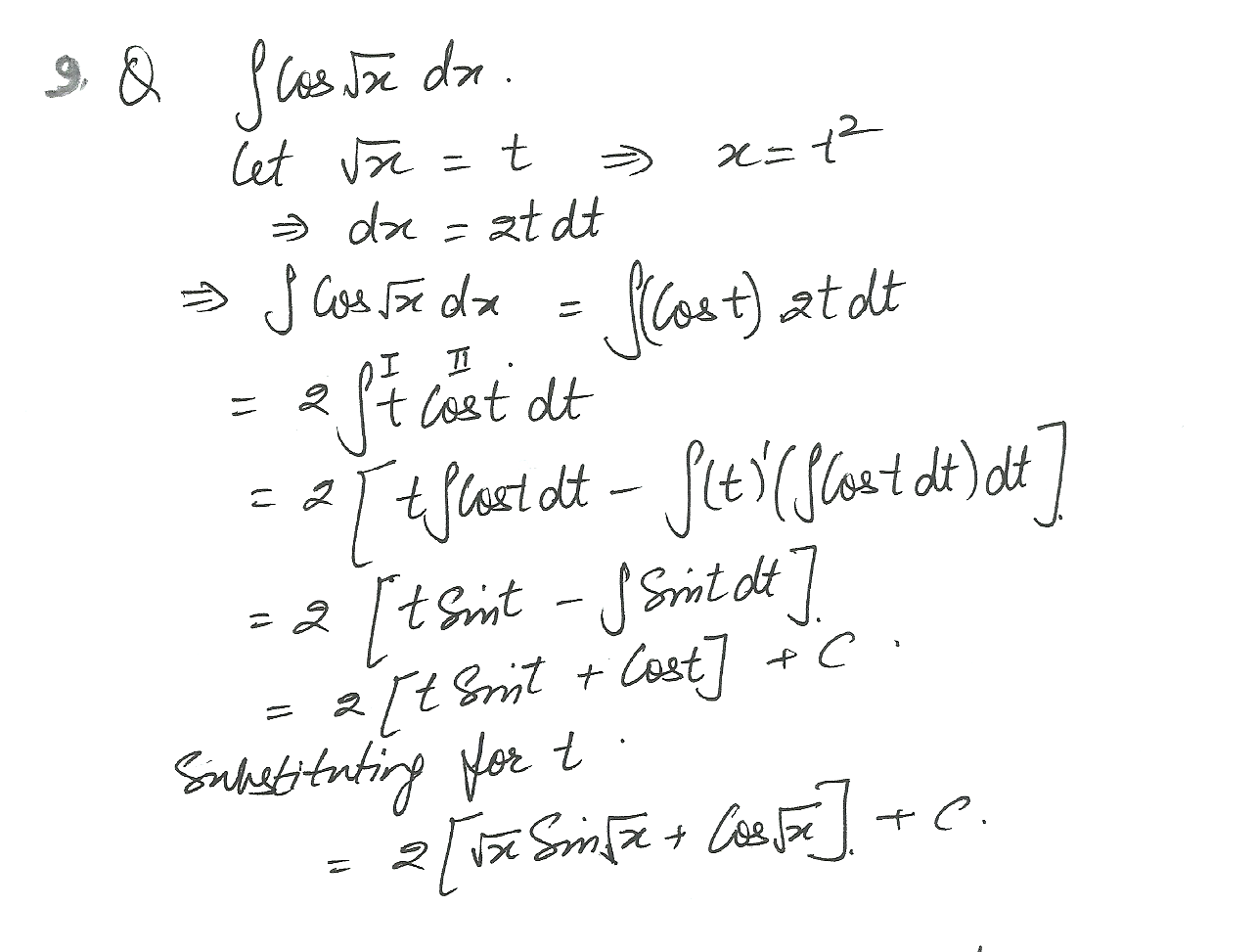5125

Mathematics Integration Level: Misc Level

Integerate the following by parts by using ILATE.
I = inverse trigonometric
L = logarithmic
A = algebraic
T = trigonometric
E = exponential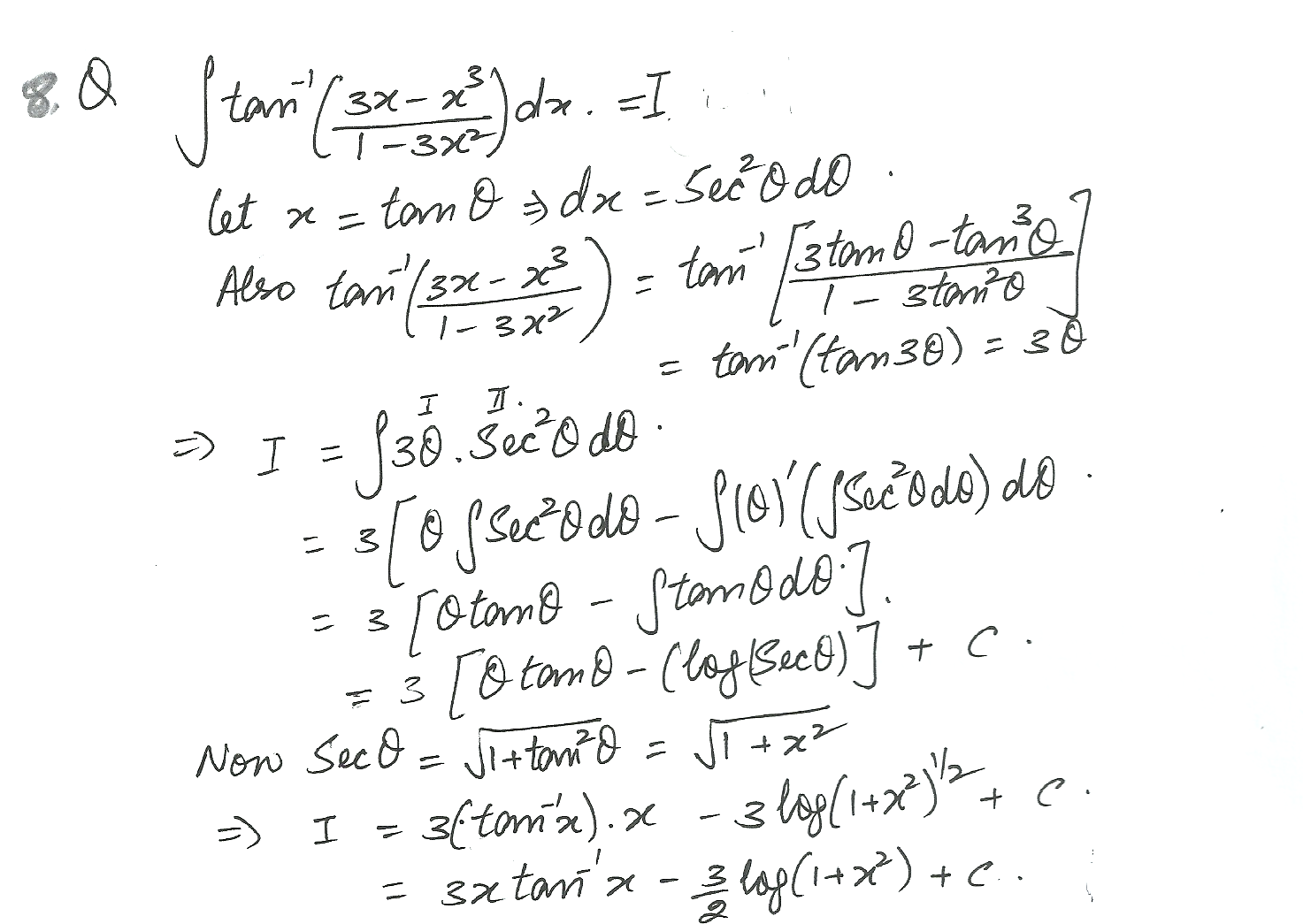5124

Mathematics Integration Level: Misc Level

Integerate the following by parts by using ILATE.
I = inverse trigonometric
L = logarithmic
A = algebraic
T = trigonometric
E = exponential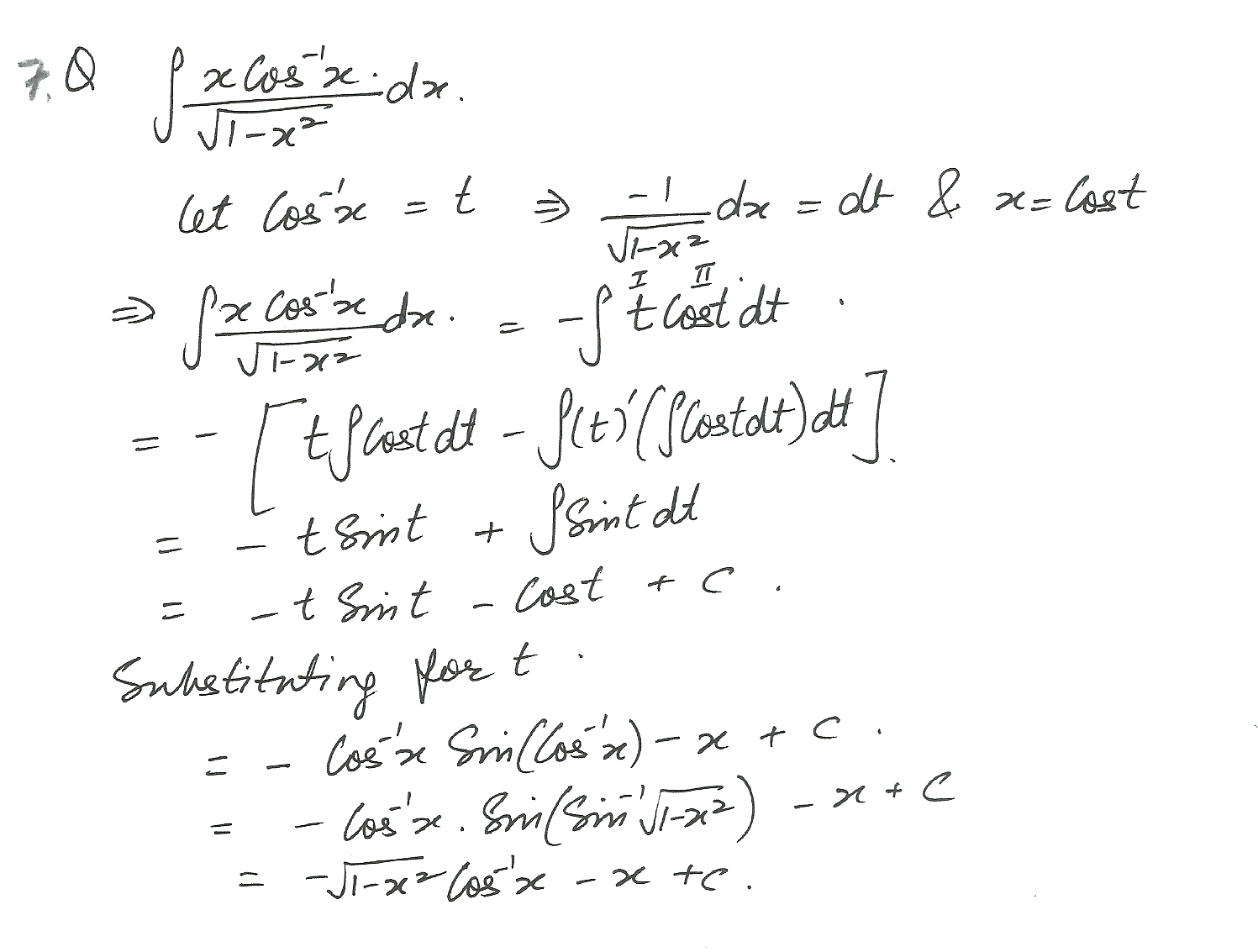5123

Mathematics Integration Level: Misc Level

Integerate the following by parts by using ILATE.
I = inverse trigonometric
L = logarithmic
A = algebraic
T = trigonometric
E = exponential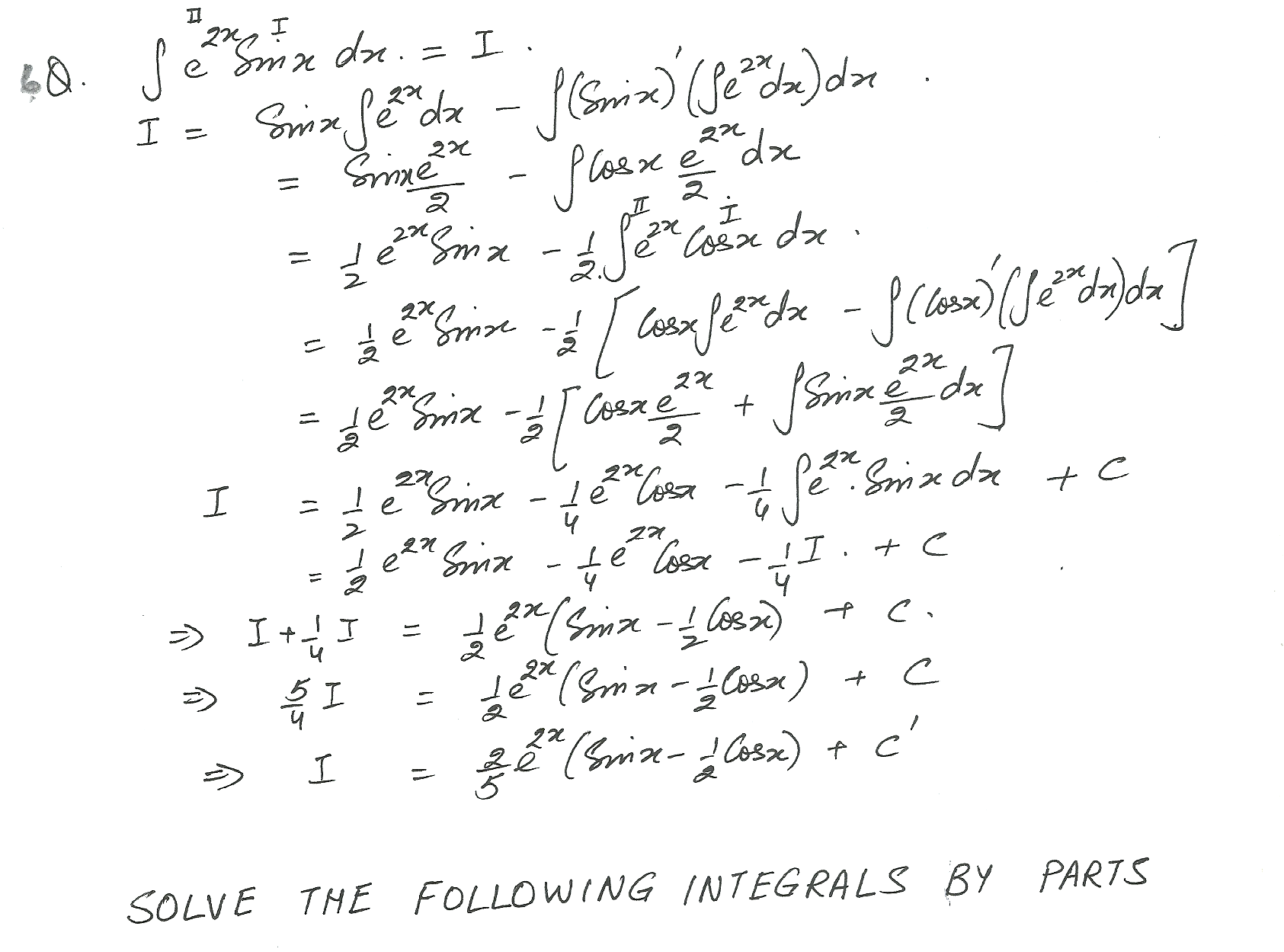5122

Mathematics Integration Level: Misc Level

Integerate the following by parts by using ILATE.
I = inverse trigonometric
L = logarithmic
A = algebraic
T = trigonometric
E = exponential5118

Mathematics Integration Level: Misc Level

Integerate the following by parts by using ILATE.
I = inverse trigonometric
L = logarithmic
A = algebraic
T = trigonometric
E = exponential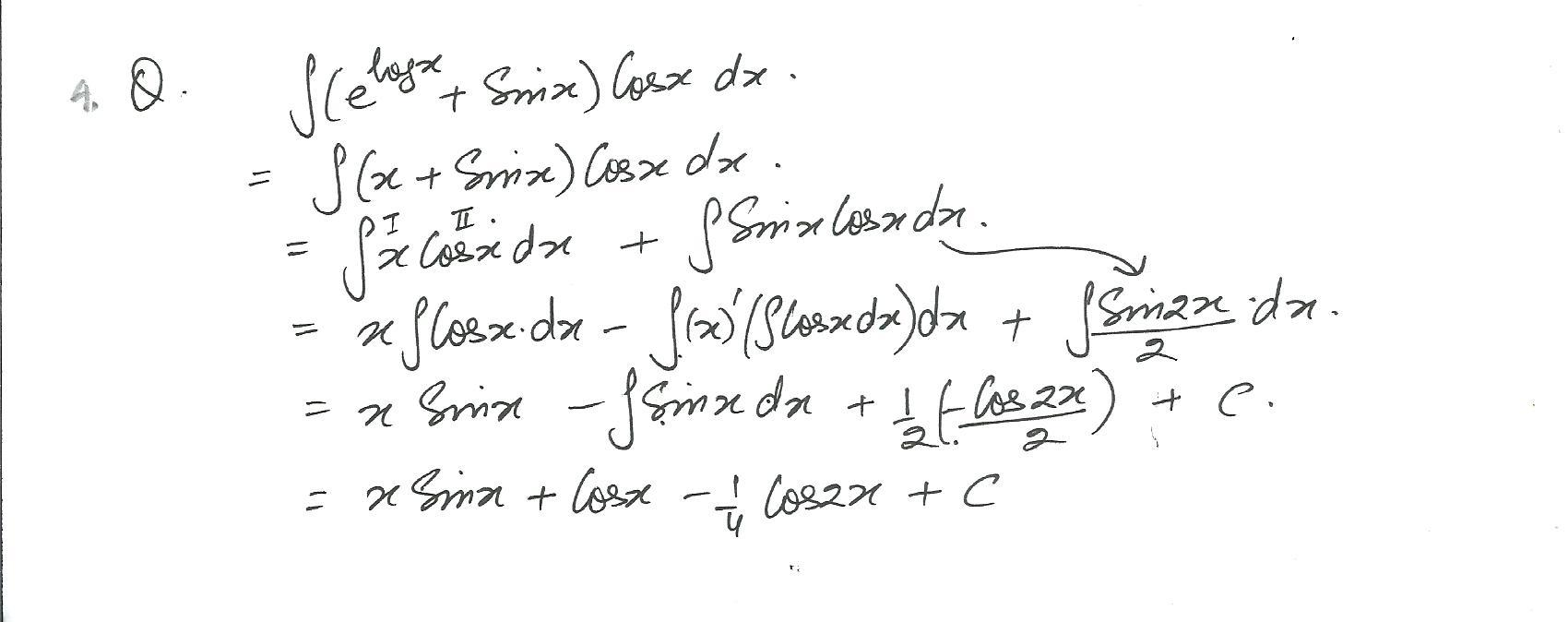5117

Mathematics Integration Level: Misc Level

Integerate the following by parts by using ILATE.
I = inverse trigonometric
L = logarithmic
A = algebraic
T = trigonometric
E = exponential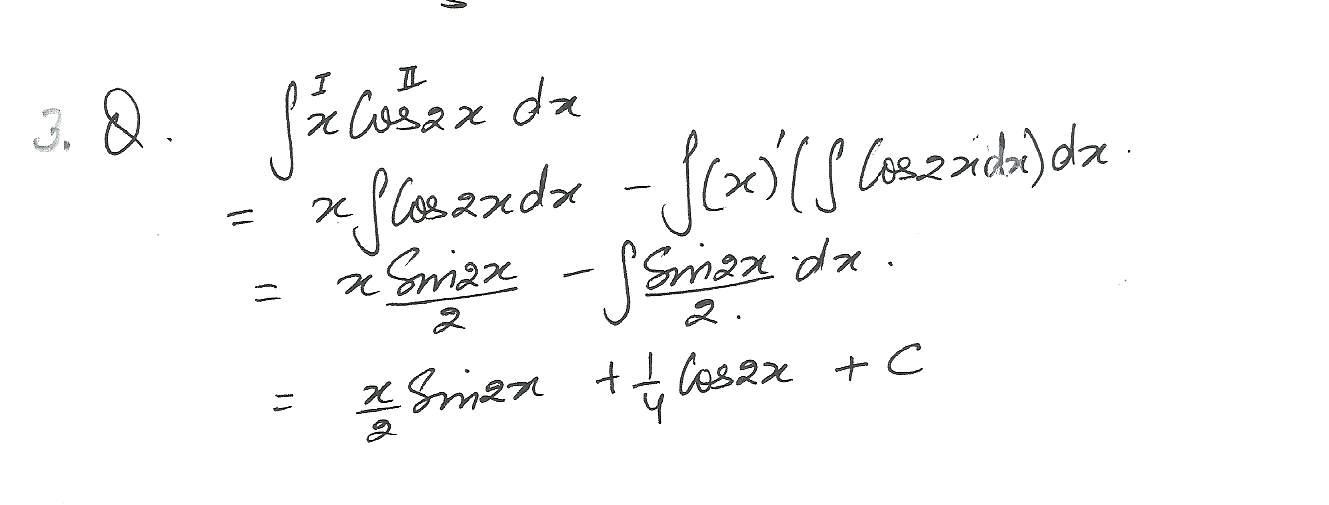5116

Mathematics Integration Level: Misc Level

Integerate the following by parts by using ILATE.
I = inverse trigonometric
L = logarithmic
A = algebraic
T = trigonometric
E = exponential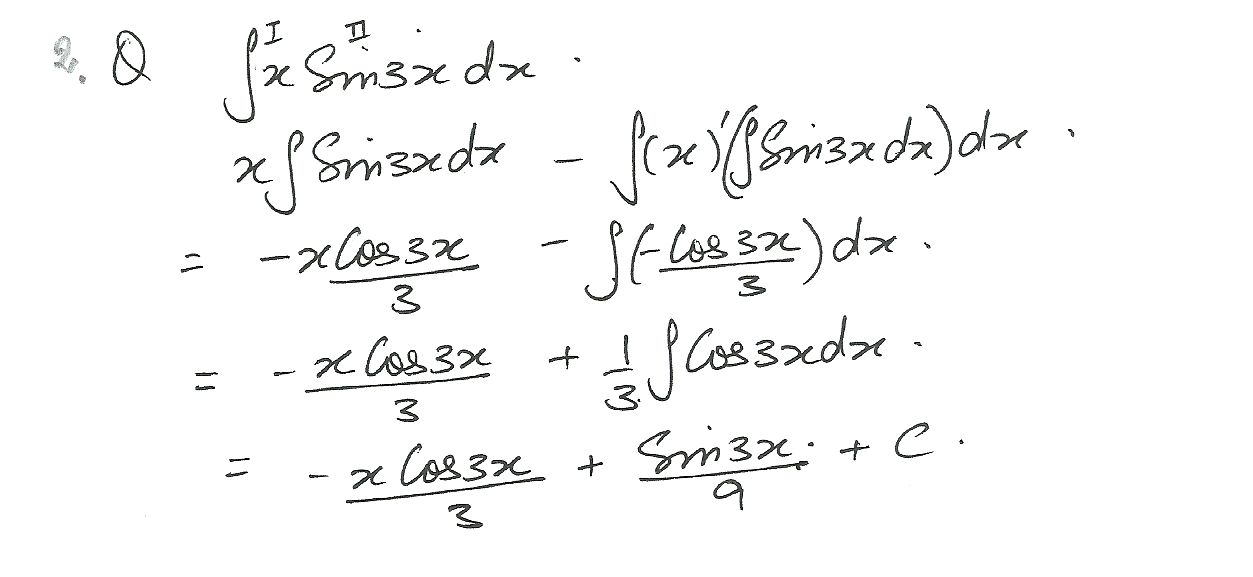5115

Mathematics Integration Level: Misc Level

Integerate the following by parts by using ILATE.
I = inverse trigonometric
L = logarithmic
A = algebraic
T = trigonometric
E = exponential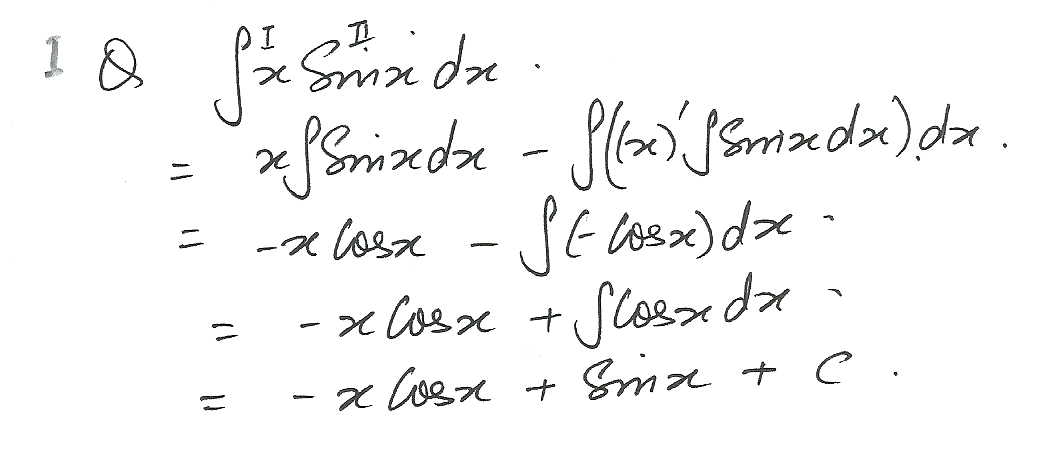4957

Mathematics Integration Level: Misc Level

Integrate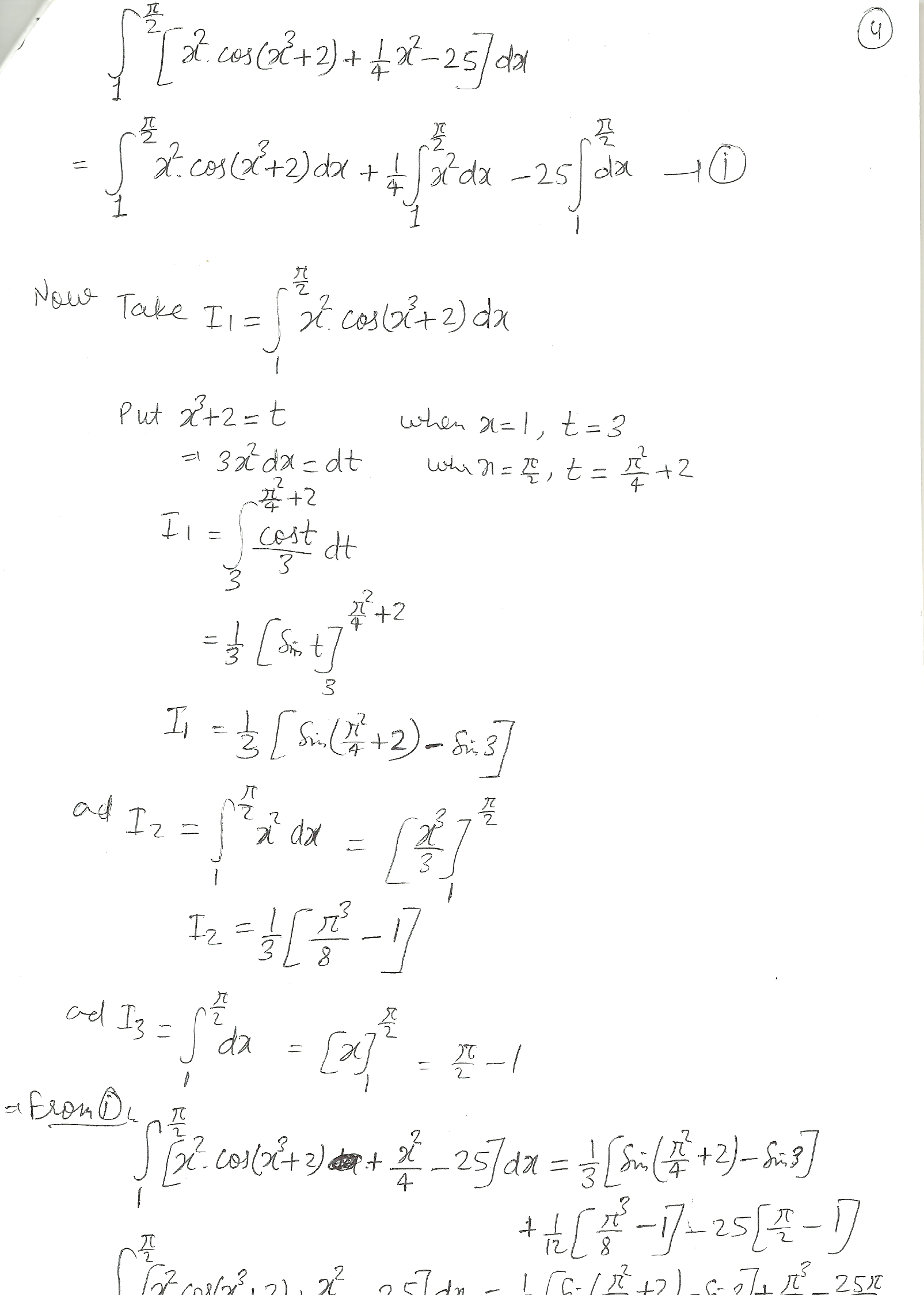4955

Mathematics Integration Level: Misc Level

Riemann sum expression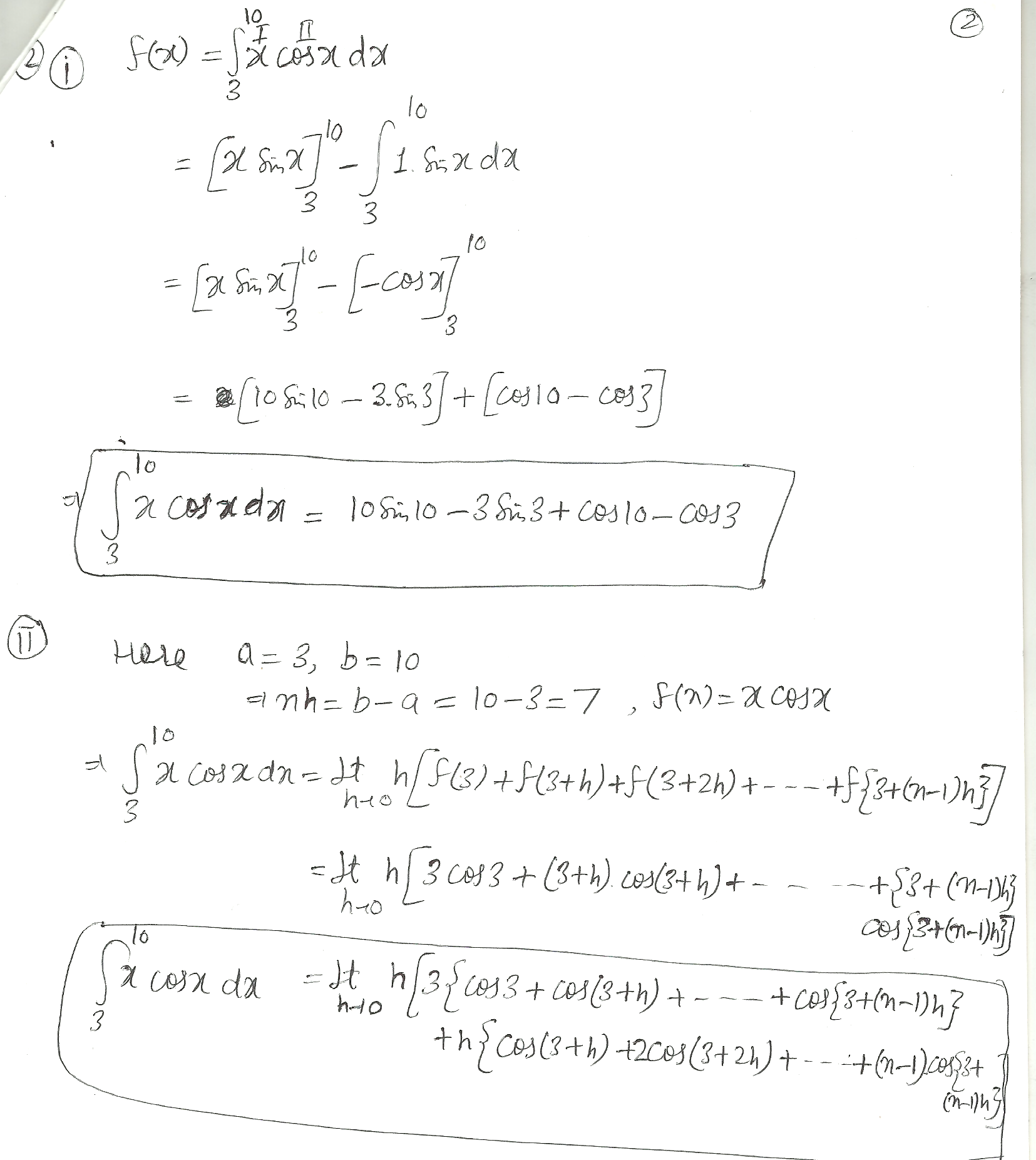4954

Mathematics Integration Level: Misc Level

Use the definition of Riemann Sum integral.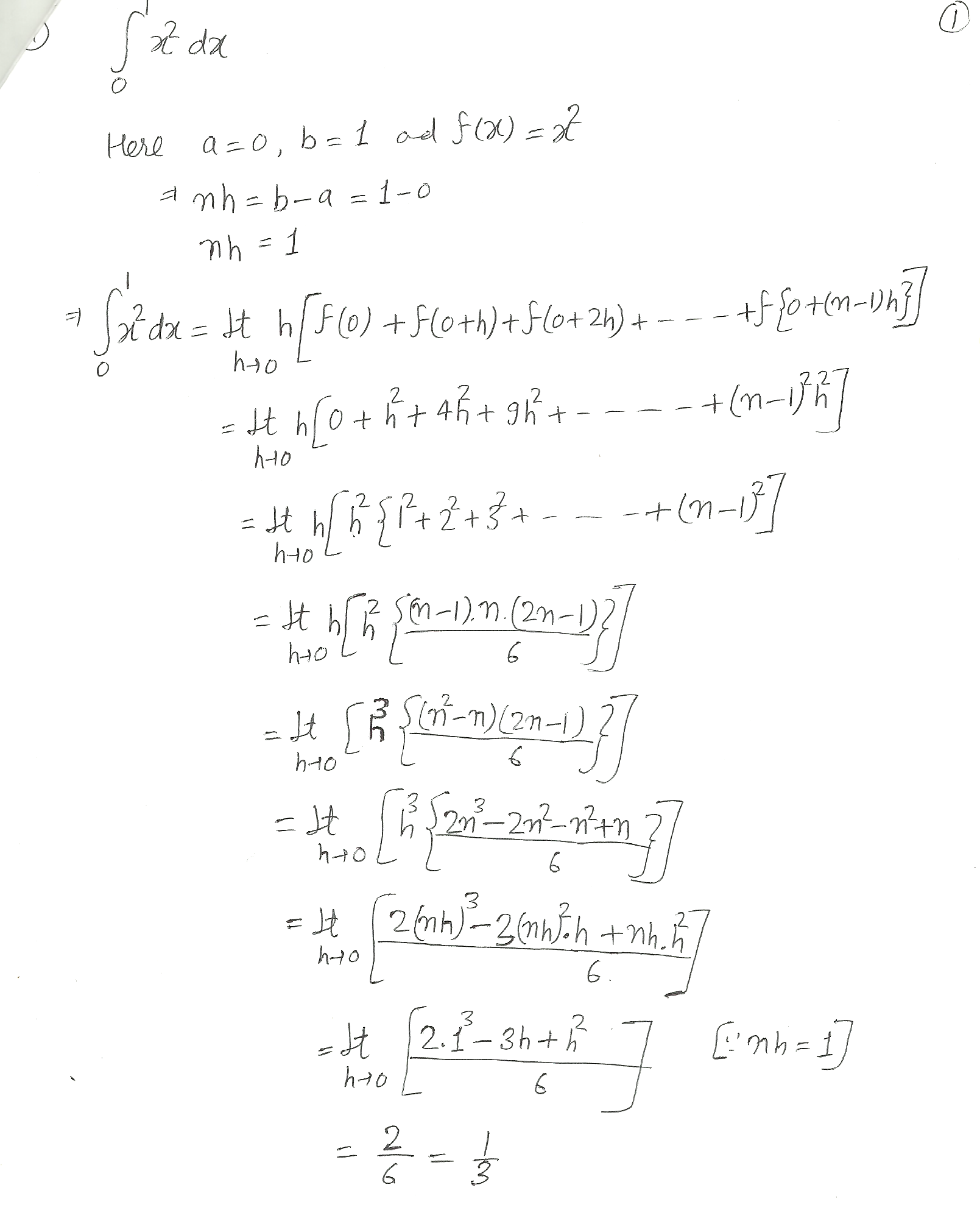Displaying 181-195 of 195 results.